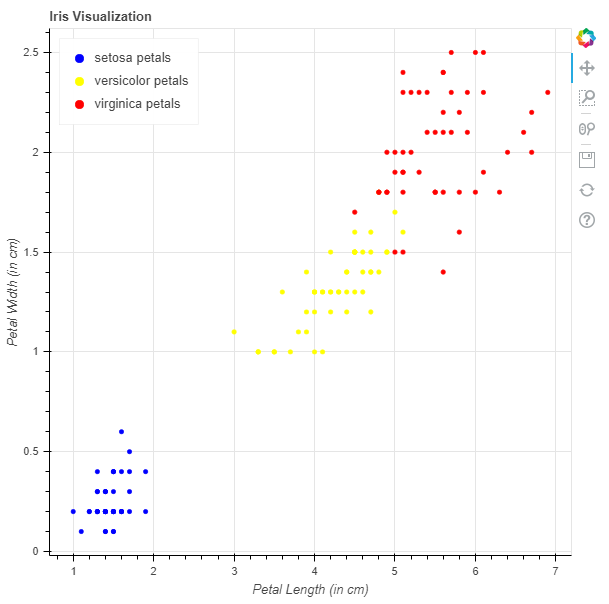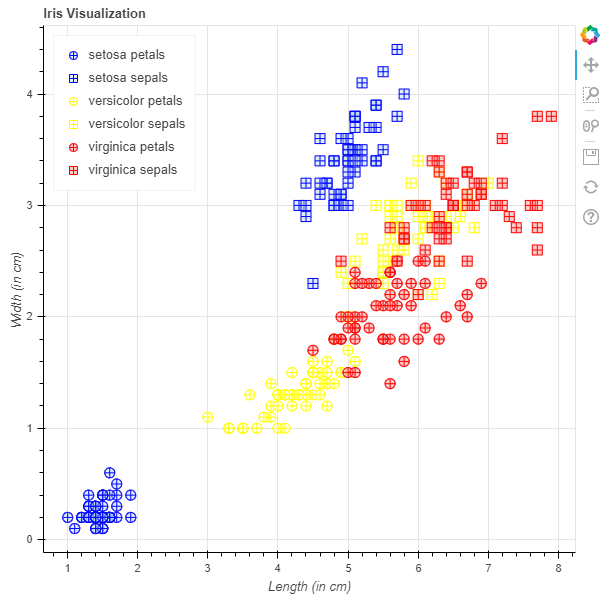Related Articles

# Python Bokeh – Visualizing the Iris Dataset

• Last Updated : 03 Jul, 2020

Bokeh is a Python interactive data visualization. It renders its plots using HTML and JavaScript. It targets modern web browsers for presentation providing elegant, concise construction of novel graphics with high-performance interactivity.

Bokeh can be used to visualize the Iris flower dataset. Visualization is be done using the `plotting` module. Here we will be using the Iris dataset given to us by Bokeh.

To download the Iris dataset run the following command on the command line :

`bokeh sampledata`

Alternatively, we can also execute the following Python code :

```import bokeh
```

## Analyzing the dataset :

In the sample data provided by Bokeh, there is a file iris.csv, this is the Iris dataset. Below is a glimpse into the iris.csv file :

```sepal_length    sepal_width    petal_length    petal_width    species
5.1        3.5        1.4        0.2        setosa
4.9        3        1.4        0.2        setosa
4.7        3.2        1.3        0.2        setosa
4.6        3.1        1.5        0.2        setosa
5        3.6        1.4        0.2        setosa
```

The dataset contains 5 attributes which are :

• sepal_length in cm
• sepal_width in cm
• petal_length in cm
• petal_width in cm
• species has 3 types of flower species :
• setosa
• versicolor
• virginica

Each species has 50 records and the total entries are 150.

## Visualizing the Dataset :

We will be plotting graphs to visualize the clustering of the data for all the 3 species.

Example 1 : Here will be plotting a graph with length of petals as the x-axis and breadth of petals as the y-axis.

1. Import the required modules :
• figure, output_file and show from bokeh.plotting
• flowers from bokeh.sampledata.iris
2. Instantiate a figure object with the title.
3. Give the names to x-axis and y-axis.
4. Plot the graphs for all the 3 species.
5. Display the model.
 `# importing the modules``from` `bokeh.sampledata.iris ``import` `flowers``from` `bokeh.plotting ``import` `figure, show, output_file`` ` `# file to save the model``output_file(``"gfg.html"``)``   ` `# instantiating the figure object``graph ``=` `figure(title ``=` `"Iris Visualization"``)`` ` `# labeling the x-axis and the y-axis``graph.xaxis.axis_label ``=` `"Petal Length (in cm)"``graph.yaxis.axis_label ``=` `"Petal Width (in cm)"`` ` `# plotting for setosa petals``x ``=` `flowers[flowers[``"species"``] ``=``=` `"setosa"``][``"petal_length"``]``y ``=` `flowers[flowers[``"species"``] ``=``=` `"setosa"``][``"petal_width"``]``color ``=` `"blue"``legend_label ``=` `"setosa petals"``graph.circle(x, y,``             ``color ``=` `color,``             ``legend_label ``=` `legend_label)`` ` `# plotting for versicolor petals``x ``=` `flowers[flowers[``"species"``] ``=``=` `"versicolor"``][``"petal_length"``]``y ``=` `flowers[flowers[``"species"``] ``=``=` `"versicolor"``][``"petal_width"``]``color ``=` `"yellow"``legend_label ``=` `"versicolor petals"``graph.circle(x, y,``             ``color ``=` `color,``             ``legend_label ``=` `legend_label)`` ` `# plotting for virginica petals``x ``=` `flowers[flowers[``"species"``] ``=``=` `"virginica"``][``"petal_length"``]``y ``=` `flowers[flowers[``"species"``] ``=``=` `"virginica"``][``"petal_width"``]``color ``=` `"red"``legend_label ``=` `"virginica petals"``graph.circle(x, y,``             ``color ``=` `color,``             ``legend_label ``=` `legend_label)`` ` `# relocating the legend table to ``# avoid abstruction of the graph``graph.legend.location ``=` `"top_left"`` ` `# displaying the model``show(graph)`

Output :Example 2 : Here will be plotting a scatter plot graph with both sepals and petals with length as the x-axis and breadth as the y-axis.

1. Import the required modules :
• figure, output_file and show from bokeh.plotting
• flowers from bokeh.sampledata.iris
2. Instantiate a figure object with the title.
3. Give the names to x-axis and y-axis.
4. Plot the graphs for all the 3 species.
5. Display the model.
 `# importing the modules``from` `bokeh.sampledata.iris ``import` `flowers``from` `bokeh.plotting ``import` `figure, show, output_file`` ` `# file to save the model``output_file(``"gfg.html"``)``   ` `# instantiating the figure object``graph ``=` `figure(title ``=` `"Iris Visualization"``)`` ` `# labeling the x-axis and the y-axis``graph.xaxis.axis_label ``=` `"Length (in cm)"``graph.yaxis.axis_label ``=` `"Width (in cm)"`` ` `# plotting for setosa petals``x ``=` `flowers[flowers[``"species"``] ``=``=` `"setosa"``][``"petal_length"``]``y ``=` `flowers[flowers[``"species"``] ``=``=` `"setosa"``][``"petal_width"``]``marker ``=` `"circle_cross"``line_color ``=` `"blue"``fill_color ``=` `"lightblue"``fill_alpha ``=` `0.4``size ``=` `10``legend_label ``=` `"setosa petals"``graph.scatter(x, y,``              ``marker ``=` `marker,``              ``line_color ``=` `line_color,``              ``fill_color ``=` `fill_color,``              ``fill_alpha ``=` `fill_alpha,``              ``size ``=` `size,``              ``legend_label ``=` `legend_label)`` ` `# plotting for setosa sepals``x ``=` `flowers[flowers[``"species"``] ``=``=` `"setosa"``][``"sepal_length"``]``y ``=` `flowers[flowers[``"species"``] ``=``=` `"setosa"``][``"sepal_width"``]``marker ``=` `"square_cross"``line_color ``=` `"blue"``fill_color ``=` `"lightblue"``fill_alpha ``=` `0.4``size ``=` `10``legend_label ``=` `"setosa sepals"``graph.scatter(x, y,``              ``marker ``=` `marker,``              ``line_color ``=` `line_color,``              ``fill_color ``=` `fill_color,``              ``fill_alpha ``=` `fill_alpha,``              ``size ``=` `size,``              ``legend_label ``=` `legend_label)`` ` `# plotting for versicolor petals``x ``=` `flowers[flowers[``"species"``] ``=``=` `"versicolor"``][``"petal_length"``]``y ``=` `flowers[flowers[``"species"``] ``=``=` `"versicolor"``][``"petal_width"``]``marker ``=` `"circle_cross"``line_color ``=` `"yellow"``fill_color ``=` `"lightyellow"``fill_alpha ``=` `0.4``size ``=` `10``legend_label ``=` `"versicolor petals"``graph.scatter(x, y,``              ``marker ``=` `marker,``              ``line_color ``=` `line_color,``              ``fill_color ``=` `fill_color,``              ``fill_alpha ``=` `fill_alpha,``              ``size ``=` `size,``              ``legend_label ``=` `legend_label)`` ` `# plotting for versicolor sepals``x ``=` `flowers[flowers[``"species"``] ``=``=` `"versicolor"``][``"sepal_length"``]``y ``=` `flowers[flowers[``"species"``] ``=``=` `"versicolor"``][``"sepal_width"``]``marker ``=` `"square_cross"``line_color ``=` `"yellow"``fill_color ``=` `"lightyellow"``fill_alpha ``=` `0.4``size ``=` `10``legend_label ``=` `"versicolor sepals"``graph.scatter(x, y,``              ``marker ``=` `marker,``              ``line_color ``=` `line_color,``              ``fill_color ``=` `fill_color,``              ``fill_alpha ``=` `fill_alpha,``              ``size ``=` `size,``              ``legend_label ``=` `legend_label)`` ` `# plotting for virginica petals``x ``=` `flowers[flowers[``"species"``] ``=``=` `"virginica"``][``"petal_length"``]``y ``=` `flowers[flowers[``"species"``] ``=``=` `"virginica"``][``"petal_width"``]``marker ``=` `"circle_cross"``line_color ``=` `"red"``fill_color ``=` `"lightcoral"``fill_alpha ``=` `0.4``size ``=` `10``legend_label ``=` `"virginica petals"``graph.scatter(x, y,``              ``marker ``=` `marker,``              ``line_color ``=` `line_color,``              ``fill_color ``=` `fill_color,``              ``fill_alpha ``=` `fill_alpha,``              ``size ``=` `size,``              ``legend_label ``=` `legend_label)`` ` `# plotting for virginica sepals``x ``=` `flowers[flowers[``"species"``] ``=``=` `"virginica"``][``"sepal_length"``]``y ``=` `flowers[flowers[``"species"``] ``=``=` `"virginica"``][``"sepal_width"``]``marker ``=` `"square_cross"``line_color ``=` `"red"``fill_color ``=` `"lightcoral"``fill_alpha ``=` `0.4``size ``=` `10``legend_label ``=` `"virginica sepals"``graph.scatter(x, y,``              ``marker ``=` `marker,``              ``line_color ``=` `line_color,``              ``fill_color ``=` `fill_color,``              ``fill_alpha ``=` `fill_alpha,``              ``size ``=` `size,``              ``legend_label ``=` `legend_label)`` ` `# relocating the legend table to ``# avoid abstruction of the graph``graph.legend.location ``=` `"top_left"``  ` `# displaying the model``show(graph)`

Output :Attention geek! Strengthen your foundations with the Python Programming Foundation Course and learn the basics.

To begin with, your interview preparations Enhance your Data Structures concepts with the Python DS Course. And to begin with your Machine Learning Journey, join the Machine Learning – Basic Level Course

My Personal Notes arrow_drop_up